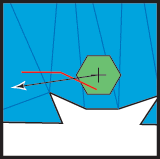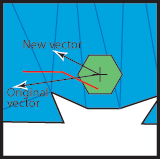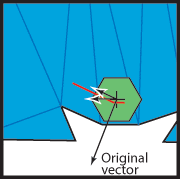PathEngine home previous: Line collision and collision info next: SlideAgainst()
Contents, Programmers Guide, Example Projects, Tutorials, Tutorial 2, Translating the agent

### Translating the agent

We use the keys 'W','S','A' and 'D' to control translation.
These move the agent forward, back, left and right respectively, relative to the current agent heading.

So the first half of the MoveAgentUnderKeyControl() function simply builds a vector from the combination of keys currently pressed, the current speed, and the current agent heading.

 ```void MoveAgentUnderKeyControl(iTestBed *testbed, iPathEngine *pathengine, iAgent *agent, float agent_heading, iCollisionContext *context, float speed, float& precisionX, float& precisionY) { double sinOf = sin(double(agent_heading)); double cosOf = cos(double(agent_heading)); double dx = precisionX; double dy = precisionY; // setup the desired movement vector in from agent heading and a combination of keys pressed if(testbed->getKeyState("W")) { // forward dx += sinOf * speed; dy += cosOf * speed; } if(testbed->getKeyState("S")) { // backward (half speed) dx -= sinOf * speed / 2; dy -= cosOf * speed / 2; } if(testbed->getKeyState("A")) { // left (half speed) dx -= cosOf * speed / 2; dy += sinOf * speed / 2; } if(testbed->getKeyState("D")) { // right (half speed) dx += cosOf * speed / 2; dy -= sinOf * speed / 2; } int32_t dxL = static_cast(dx); int32_t dyL = static_cast(dy); if(dxL == 0 && dyL == 0) { // no movement after approximation precisionX = static_cast(dx); precisionY = static_cast(dy); return; } //... implement the requested movement } ```

To implement this movement we go through iAgent::firstCollisionTo().
If this returns zero then we know we can move without collision.
Otherwise it returns an iCollsionInfo object from which we will obtain information about the obstruction we want to slide against.

Passing a collision context into firstCollisionTo() means that the collision will take account of any obstacles that have been placed (and added to the context).

 ```cPosition current = agent->getPosition(); double targetX = current.x + dx; double targetY = current.y + dy; cCollidingLine l; unique_ptr collidingAgent; cPosition target; target.x = static_cast(targetX); target.y = static_cast(targetY); bool collides = agent->firstCollisionTo(context, target.x, target.y, target.cell, l, collidingAgent); int trys = 0; while(collides && trys < 2) { int32_t coords; coords = l.startX; coords = l.startY; coords = l.endX; coords = l.endY; SlideAgainst(coords, current.x, current.y, targetX, targetY); target.x = static_cast(targetX); target.y = static_cast(targetY); collides = agent->firstCollisionTo(context, target.x, target.y, target.cell, l, collidingAgent); trys++; } if(collides) { // failed to find a non-colliding movement vector return; } // succeeded precisionX = static_cast(targetX - target.x); precisionY = static_cast(targetY - target.y); agent->moveTo(target); ```

The obstruction edge is obtained from the iCollisionInfo object as an array of four longs.
This corresponds to <startx,starty,endx,endy> for a 2d obstructing line.
This obstructing line comes from the expanded geometry, and so is relative to the agent centre.SlideAgainst() is defined in 'sliding.cpp'.
This function modifies a movement vector to slide against an obstruction edge.Note that for simplicity we don't make any attempt to move the agent right up to the point of contact.

Sometimes it is necessary to slide against two edges in order to get the correct behaviour at convex corners.
Two tries seems to be sufficient for most cases.Documentation for PathEngine release 6.03 - Copyright © 2002-2021 PathEngine next: SlideAgainst()